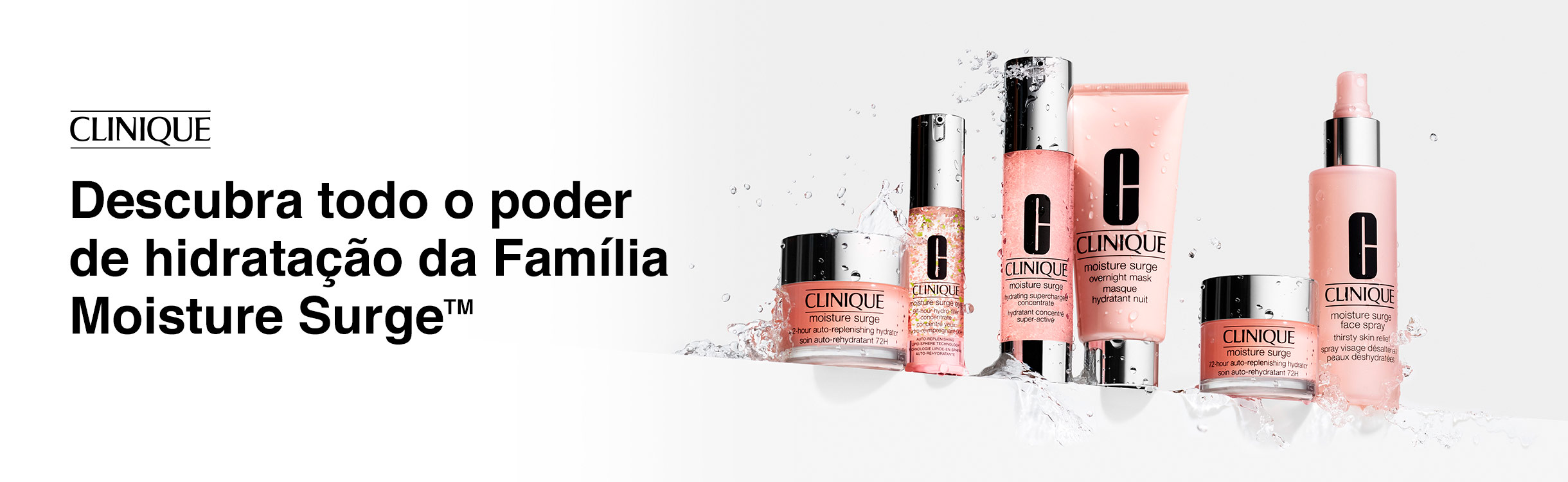# Clinique Beyond Perfecting

Clinique Beyond Perfecting traz cobertura para o rosto todo com fórmula leve. Beyond Perfecting conta com bases e corretivos faciais que proporcionam duração de mais de 12 horas na pele e são à prova d’água.

## Todos os Produtos

-27%R\$ 339,90
R\$ 245,90
8x de R\$ 30,74
Frete GrátisR\$ 339,90
10x de R\$ 33,99
Frete GrátisR\$ 339,90
10x de R\$ 33,99
Frete GrátisR\$ 339,90
10x de R\$ 33,99
Frete Grátis
-8%R\$ 244,90
R\$ 222,90
7x de R\$ 31,84
Frete GrátisR\$ 339,90
10x de R\$ 33,99
Frete Grátis
-7%R\$ 339,90
R\$ 315,90
10x de R\$ 31,59
Frete GrátisR\$ 339,90
10x de R\$ 33,99
Frete Grátis
-15%R\$ 339,90
R\$ 285,90
9x de R\$ 31,77
Frete GrátisR\$ 339,90
10x de R\$ 33,99
Frete Grátis
-6%R\$ 339,90
R\$ 317,90
10x de R\$ 31,79
Frete Grátis
-15%R\$ 244,90
R\$ 206,90
6x de R\$ 34,48
Frete Grátis
-14%R\$ 244,90
R\$ 208,90
6x de R\$ 34,82
Frete Grátis
-27%R\$ 339,90
R\$ 245,90
8x de R\$ 30,74
Frete GrátisR\$ 339,90
10x de R\$ 33,99
Frete GrátisR\$ 198,90
6x de R\$ 33,15
Frete Grátis
-15%R\$ 339,90
R\$ 287,90
9x de R\$ 31,99
Frete GrátisR\$ 278,90
9x de R\$ 30,99
Frete GrátisR\$ 339,90
10x de R\$ 33,99
Frete GrátisR\$ 339,90
10x de R\$ 33,99
Frete GrátisR\$ 339,90
10x de R\$ 33,99
Frete GrátisR\$ 339,90
10x de R\$ 33,99
Frete GrátisR\$ 339,90
10x de R\$ 33,99
Frete GrátisR\$ 339,90
10x de R\$ 33,99
Frete GrátisR\$ 339,90
10x de R\$ 33,99
Frete GrátisR\$ 339,90
10x de R\$ 33,99
Frete GrátisR\$ 339,90
10x de R\$ 33,99
Frete GrátisR\$ 339,90
10x de R\$ 33,99
Frete GrátisR\$ 288,90
9x de R\$ 32,10
Frete GrátisR\$ 244,90
8x de R\$ 30,61
Frete GrátisR\$ 278,90
9x de R\$ 30,99
Frete GrátisR\$ 339,90
10x de R\$ 33,99
Frete GrátisR\$ 339,90
10x de R\$ 33,99
Frete GrátisR\$ 224,90
7x de R\$ 32,13
Frete GrátisR\$ 339,90
10x de R\$ 33,99
Frete Grátis
Você está offline.